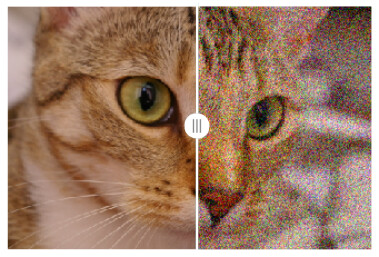# Brainstorming ideas: Better Overlay Experience in Jupyter Notebooks

While iterating on a segmentation workflow, it is good to have a previous/after view.
Currently, that can be done with an overlay or displaying the pictures left and right.
I just want to propose some ideas that would make it more interactive to explore data.
Here are some conceptual ideas:
Left-Right-Slider:Showing a round frame when hovering with the mouse:

Same, but, opacity of second layer reduces Gaussian-like) when hovering with the mouse:

Here is how the code could look like:

``````from skimage import data
from skimage.util import random_noise
import matplotlib.pyplot as plt
import numpy as np

img = data.chelsea()
noisy_img = random_noise(img, var=0.02)

fig1= plt.figure()
plt.imshow(img)
plt.axis('off')
fig2= plt.figure()
plt.imshow(noisy_img)
plt.axis('off')

#new part (does not exist yet):
from splitview import SplitMapControl
control = SplitMapControl(left_layer=fig1, right_layer=fig2)
``````

I don’t want to suggest that this should be a feature of itk, I just want to share this idea, that might be very useful in the Jupyter environment for image analysis.
Maybe this can become a Jupyter widget one day.

And this is the code to reproduce the two mouse-hover examples:

``````from skimage import data
import matplotlib.pyplot as plt
import numpy as np
import cv2
plt.rcParams['image.cmap']='gray'
def rgb2gray(rgb):
return np.dot(rgb[...,:3], [0.2989, 0.5870, 0.1140])

img = data.chelsea()
bw_img = rgb2gray(img)
rgba = cv2.cvtColor(img, cv2.COLOR_RGB2RGBA)
pixY,pixX , _ = img.shape

# disk
x, y = np.ogrid[0:pixY, 0:pixX]
x, y
mask = (x - pixY/2) ** 2 + (y - pixX/2) ** 2 > 100 ** 2

rgba = cv2.cvtColor(img, cv2.COLOR_RGB2RGBA)

print((rgba.shape))
plt.axis('off')
plt.imshow(bw_img)
plt.imshow(rgba)

# gauss
x, y = np.meshgrid(np.linspace(-1,1,pixX), np.linspace(-1,1,pixY))
d = np.sqrt(x**2+(y*0.78)**2)
sigma, mu = 0.4, 0
g = 255*np.exp(-( (d-mu)**2 / ( 2.0 * sigma**2 ) ) )

rgba[:,:,3]=g
print((rgba[0,0]))
plt.figure()
plt.axis('off')
plt.imshow(bw_img)
plt.imshow(rgba)
``````
3 Likes